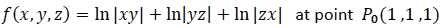# Derivative of directional vector

Gold Member

## Homework Statement

Find the unit vectors along which the given functions below increase and decrease most rapidly at P0 . Then find the derivatives of the functions in these directions.## Homework Equations

solution:## The Attempt at a Solution

why are the derivatives’ values along these two directions are 2*sqrt(3)?
as (∇f)(P0)= <1,1,1>, absolute value of it = sqrt(3)
why is the solution multiplied 2 for it?
thanks

#### Attachments

Staff Emeritus
Homework Helper
Gold Member
as (∇f)(P0)= <1,1,1>,
It isn’t. Double check your computations.

Homework Helper
Dearly Missed

## Homework Statement

Find the unit vectors along which the given functions below increase and decrease most rapidly at P0 . Then find the derivatives of the functions in these directions.

View attachment 213475

## Homework Equations

solution:
View attachment 213474

## The Attempt at a Solution

why are the derivatives’ values along these two directions are 2*sqrt(3)?
as (∇f)(P0)= <1,1,1>, absolute value of it = sqrt(3)
why is the solution multiplied 2 for it?
thanks

You are correct: the directional derivative is, indeed, ##2 \sqrt{3}##. The reason is that ##\nabla f(1,1,1) = \langle 2,2,2 \rangle## is a vector of length ##\sqrt{3 \times 4} = 2 \sqrt{3}##.

Staff Emeritus